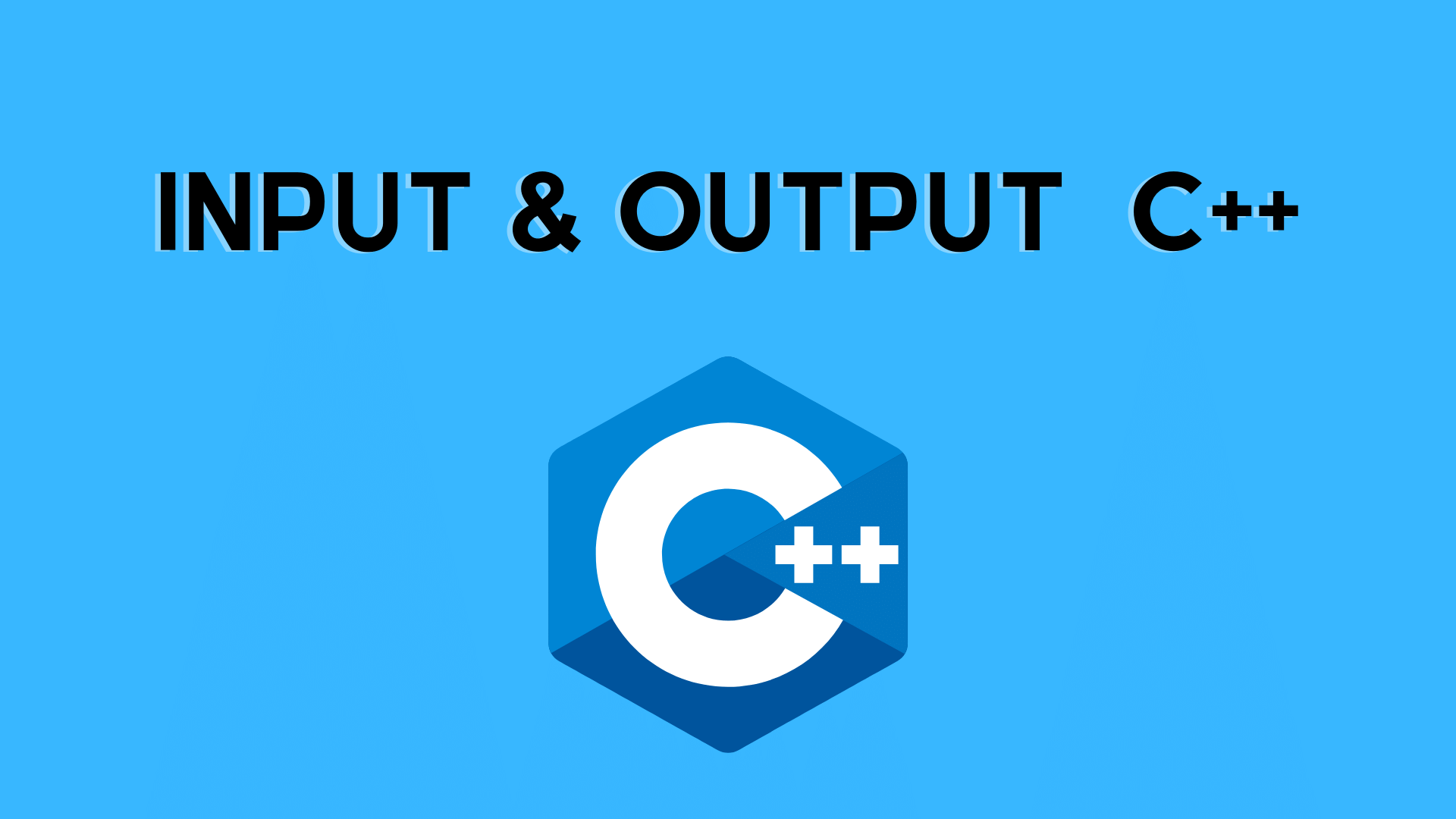## Input and Output in C++ | C++ Programming

In this tutorial, you will learn about Basic Input/Output in C++

With the use of examples, we will learn how to utilize the cin object to take user input and the cout object to display output to the user in this tutorial.

input-output in c input-output in c input-output in c input-output
Output in C++
cout is a C++ function that transmits formatted output to standard output devices like the screen. For displaying output, we utilize the cout object in conjunction with the operator.

Ex. 1: String Output

```#include <iostream>
using namespace std;

int main() {
// prints the string enclosed in double quotes
cout << "Developer's Dome";
return 0;
}```

Output

Developer’s Dome

## What is the procedure for implementing this program?

• The iostream header file, which allows us to display output, is initially included.
• Within the std namespace, the cout object is defined. We used the using namespace std; statement to use the std namespace.
• The main() function is the first function in any C++ programme. The execution of the code begins with the main() function.
• The object cout prints the string enclosed in quotation marks ” “. It’s followed by the operator. return 0; is the main() function’s “exit status.” This statement comes at the end of the programme, but it is not required.
```#include <iostream>

int main() {
// prints the string enclosed in double quotes
std::cout << "without use of namespace";
return 0;
}
```

Output

without use of namespace

## Numbers and Character’s Output

We use the same cout object to output the numbers and character variables, but without quotation marks.

```#include <iostream>
using namespace std;

int main() {
int num1 = 70;
double num2 = 256.783;
char ch = 'A';

cout << num1 << endl;    // print integer
cout << num2 << endl;    // print double
cout << "character: " << ch << endl;    // print char
return 0;
}
```

Output

70
256.783
character: A

Input in C++

cin accepts structured input from standard input devices like the keyboard in C++. For taking input, we use the cin object and the >> operator.

```#include <iostream>
using namespace std;

int main() {
int num;
cout << "Enter an integer: ";
cin >> num;   // Taking input
cout << "The number is: " << num;
return 0;
}
```

Output

Enter an integer: 70
The number is: 70

## C++ Taking Multiple Inputs

```#include <iostream>
using namespace std;

int main() {
char a;
int num;

cout << "Enter a character and an integer: ";
cin >> a >> num;

cout << "Character: " << a << endl;
cout << "Number: " << num;

return 0;
}```

Output

Enter a character and an integer: F
23
Character: F
Number: 23

You may like:

Features of C++ | C++ Tutorial

Introduction to C++ Language

C++ Compiler